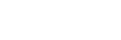Explore Kits

# Cricket match booking game on Python

by jatinrdx606 Updated: Nov 27, 2022

import random

import math

import csv

# Cricket Ticket Manegment booking

# creater : JATIN

#main data detail of price and match

DATE=input("Enter Date DD/MM/YYYY :\n")

match=input("Match Name :\n")

country1=input("Enter 1st  Team Country Name :\n")

country2=input("Enter 2st  Team Country Name :\n")

price=int(input("One Ticket Price"))

print("Details are Fill Succesfully")

# while loop

user=int(input("How much seats Need Maximum 6  :\n"))        #max 6 seats book one time

pay=input("1.Online\n2.Cash\n3.Other\n4.Type :\n")

print("Back Booking Press 9")

def paying(pay):

if pay==3:

pay="Other"

elif pay==1:

pay="Online"

if pay==2:

pay="Cash"

else:

print("Try Again")

#sender user val

def amountTicket():

if user==1:

print("1 Ticket")

code1=random.randint(000,9999999)

Ticket1=input("Enter Name : ")

mobilenumber1=input("Mobile Number :\n")

print("Successfully Ticket Book\n\n")

print("Name: ",Ticket1,  match,    DATE)

print(country,code1,country1," Vs ",country2)

print(pay,  "Amount"   ,price)

elif user==2:

print("1 Ticket")

code1=random.randint(000,9999999)

Ticket1=input("Enter Name : ")

mobilenumber1=input("Mobile Number :\n")

print("\n2 Ticket")

code2=random.randint(000,9999999)

Ticket2=input("Enter Name : ")

mobilenumber2=input("Mobile Number :\n")

print("Successfully Ticket Book\n\n")

print("Name: ",Ticket1,  match,    DATE)

print(country,code1,country1," Vs ",country2)

print(pay,  "Amount"   ,price)

print("\n 2nd Ticket")

print("Name: ",Ticket2,  match,    DATE)

print(country,code2,country1," Vs ",country2)

print(pay,  "Amount"   ,price)

print("\n\nTotal 2 Ticket",price*user)

if user==3:

print("1 Ticket")

code1=random.randint(000,9999999)

Ticket1=input("Enter Name : ")

mobilenumber1=input("Mobile Number :\n")

print("\n2 Ticket")

code2=random.randint(000,9999999)

Ticket2=input("Enter Name : ")

mobilenumber2=input("Mobile Number :\n")

print("\n3 Ticket")

code3=random.randint(000,9999999)

Ticket3=input("Enter Name : ")

mobilenumber3=input("Mobile Number :\n")

print("Successfully Ticket Book\n\n")

print("\n1 Ticket")

print("Name: ",Ticket1,  match,    DATE)

print(country,code1,country1," Vs ",country2)

print(pay,  "Amount"   ,price)

print("\n 2nd Ticket")

print("Name: ",Ticket2,  match,    DATE)

print(country,code2,country1," Vs ",country2)

print(pay,  "Amount"   ,price)

print("\n 3nd Ticket")

print("Name: ",Ticket3,  match,    DATE)

print(country,code3,country1," Vs ",country2)

print(pay,  "Amount"   ,price)

print("\n\nTotal 3 Ticket",price*user)

elif user==4:

print("1 Ticket")

code1=random.randint(000,9999999)

Ticket1=input("Enter Name : ")

mobilenumber1=input("Mobile Number :\n")

print("\n2 Ticket")

code2=random.randint(000,9999999)

Ticket2=input("Enter Name : ")

mobilenumber2=input("Mobile Number :\n")

print("\n3 Ticket")

code3=random.randint(000,9999999)

Ticket3=input("Enter Name : ")

mobilenumber3=input("Mobile Number :\n")

print("\n4 Ticket")

code4=random.randint(000,9999999)

Ticket4=input("Enter Name : ")

mobilenumber4=input("Mobile Number :\n")

print("Successfully Ticket Book\n\n")

print("\n1 Ticket")

print("Name: ",Ticket1,  match,    DATE)

print(country,code1,country1," Vs ",country2)

print(pay,  "Amount"   ,price)

print("\n 2nd Ticket")

print("Name: ",Ticket2,  match,    DATE)

print(country,code2,country1," Vs ",country2)

print(pay,  "Amount"   ,price)

print("\n 3nd Ticket")

print("Name: ",Ticket3,  match,    DATE)

print(country,code3,country1," Vs ",country2)

print(pay,  "Amount"   ,price)

print("\n 4nd Ticket")

print("Name: ",Ticket4,  match,    DATE)

print(country,code4,country1," Vs ",country2)

print(pay,  "Amount"   ,price)

print("\n\nTotal 4 Ticket",price*user)

if user==5:

print("1 Ticket")

code1=random.randint(000,9999999)

Ticket1=input("Enter Name : ")

mobilenumber1=input("Mobile Number :\n")

print("\n2 Ticket")

code2=random.randint(000,9999999)

Ticket2=input("Enter Name : ")

mobilenumber2=input("Mobile Number :\n")

print("\n3 Ticket")

code3=random.randint(000,9999999)

Ticket3=input("Enter Name : ")

mobilenumber3=input("Mobile Number :\n")

print("\n4 Ticket")

code4=random.randint(000,9999999)

Ticket4=input("Enter Name : ")

mobilenumber4=input("Mobile Number :\n")

print("\n5 Ticket")

code5=random.randint(000,9999999)

Ticket5=input("Enter Name : ")

mobilenumber5=input("Mobile Number :\n")

print("Successfully Ticket Book\n\n")

print("\n1 Ticket")

print("Name: ",Ticket1,  match,    DATE)

print(country,code1,country1," Vs ",country2)

print(pay,  "Amount"   ,price)

print("\n 2nd Ticket")

print("Name: ",Ticket2,  match,    DATE)

print(country,code2,country1," Vs ",country2)

print(pay,  "Amount"   ,price)

print("\n 3nd Ticket")

print("Name: ",Ticket3,  match,    DATE)

print(country,code3,country1," Vs ",country2)

print(pay,  "Amount"   ,price)

print("\n 4nd Ticket")

print("Name: ",Ticket4,  match,    DATE)

print(country,code4,country1," Vs ",country2)

print(pay,  "Amount"   ,price)

print("\n 5nd Ticket")

print("Name: ",Ticket5,  match,    DATE)

print(country,code5,country1," Vs ",country2)

print(pay,  "Amount"   ,price)

print("\n\nTotal 5 Ticket",price*user)

if user==6:

print("1 Ticket")

code1=random.randint(000,9999999)

Ticket1=input("Enter Name : ")

mobilenumber1=input("Mobile Number :\n")

print("\n2 Ticket")

code2=random.randint(000,9999999)

Ticket2=input("Enter Name : ")

mobilenumber2=input("Mobile Number :\n")

print("\n3 Ticket")

code3=random.randint(000,9999999)

Ticket3=input("Enter Name : ")

mobilenumber3=input("Mobile Number :\n")

print("\n4 Ticket")

code4=random.randint(000,9999999)

Ticket4=input("Enter Name : ")

mobilenumber4=input("Mobile Number :\n")

print("\n5 Ticket")

code5=random.randint(000,9999999)

Ticket5=input("Enter Name : ")

mobilenumber5=input("Mobile Number :\n")

print("\n6 Ticket")

code6=random.randint(000,9999999)

Ticket6=input("Enter Name : ")

mobilenumber6=input("Mobile Number :\n")

print("Successfully Ticket Book\n\n")

print("\n1 Ticket")

print("Name: ",Ticket1,  match,    DATE)

print(country,code1,country1," Vs ",country2)

print(pay,  "Amount"   ,price)

print("\n 2nd Ticket")

print("Name: ",Ticket2,  match,    DATE)

print(country,code2,country1," Vs ",country2)

print(pay,  "Amount"   ,price)

print("\n 3nd Ticket")

print("Name: ",Ticket3,  match,    DATE)

print(country,code3,country1," Vs ",country2)

print(pay,  "Amount"   ,price)

print("\n 4nd Ticket")

print("Name: ",Ticket4,  match,    DATE)

print(country,code4,country1," Vs ",country2)

print(pay,  "Amount"   ,price)

print("\n 5nd Ticket")

print("Name: ",Ticket5,  match,    DATE)

print(country,code5,country1," Vs ",country2)

print(pay,  "Amount"   ,price)

print("\n 6nd Ticket")

print("Name: ",Ticket6,  match,    DATE)

print(country,code6,country1," Vs ",country2)

print(pay,  "Amount"   ,price)

print("\n\nTotal 6 Ticket",price*user)

amountTicket()

Open Weaver – Develop Applications Faster with Open Source

Follow

• © 2023 Open Weaver Inc.